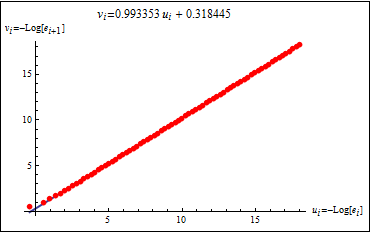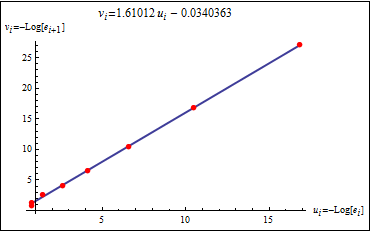# Tutorial: assessing convergence of nonlinear solvers

Given software that finds a value of x that makes$f(x)=0$, how do you infer the rate of convergence of the algorithm embedded in the software?  The answer is to do some tests for which you know the answer.  Shown below are convergence plots of the error$\left(e_i = |x_i-x^\text{exact}|\right)$ for three solver methods applied to find a zero of the function$f(x)=(x-1.5)(x-2.3)$. In all cases, the first guess is taken so that the root$x=1.5$ is found by the solvers. The errors at each iteration are used to generate points on a convergence plot as indicated.  The slope of the plot is the rate of convergence. The zip file, 5newtonIterationErrorConvergenceAnalysis.zip, contains the Mathematica commands (.pdf and .nb) used to conduct this study.

(a) Classical Newton-RaphsonClassical Newton-Raphson iteration, here applied to find the zero of the function (x-1.5)(x-2.3) using a starting guess of x=0, has approximately second-order convergence (slope of the line).

(b) Modified Newton-Raphson:The modified Newton-Raphson method, which uses the function slope at the first iteration for all subsequent iterations, has approximately first-order convergence and thus requires more iterations (more red dots).

(c) Secant solver:Convergence for a secant solver, in which the function slope is approximated by the secant connecting two first guesses (x=0 and x=0.5), showing a convergence rate (slope of this line) somewhere between 1st-order and 2nd-order

The zip file, 5newtonIterationErrorConvergenceAnalysis.zip, contains the Mathematica commands (.pdf and .nb) used to conduct this study.